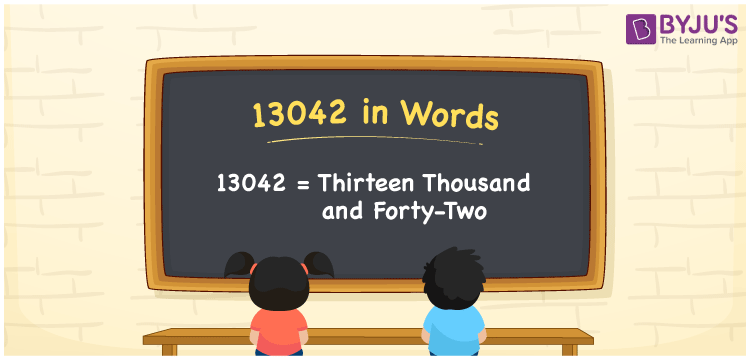# 13042 in Words

13042 in words is written as Thirteen thousand forty-two. In both the International System of Numerals and the Indian System of Numerals, 13042 is written as Thirteen thousand forty-two. The number 13042 is a Cardinal Number as it denotes some quantity. For example, “that mobile phone costs 13042 rupees”.

 13042 in Words Thirteen thousand forty-two Thirteen thousand forty-two in Number 13042

## 13042 in English Words

13042 in English words is read as “Thirteen thousand forty-two”.## How to Write 13042 in Words?

To write 13042 in words, we shall use the place value chart. In the place value chart, put 1 in the ten thousands, 3 in the thousands, 0 in the hundreds, 4 in the tens and 2 in the ones. Let us make a place value chart to write the number 13042 in words.

 Ten Thousands Thousands Hundreds Tens Ones 1 3 0 4 2

Thus, we can write the expanded form as

1 × Ten Thousand + 3 × Thousand + 0 × Hundred + 4 × Ten + 2 × One

= 1 × 10000 + 3 × 1000 + 0 × 100 + 4 × 10 + 2 × 1

= 10000 + 3000 + 0 +40 + 2

= 13042

= Thirteen thousand forty-two.

13042 is a natural number, the successor of 13041 and the predecessor of 13043.

13042 in words – Thirteen thousand forty-two

• Is 13042 an odd number? – No
• Is 13042 an even number? – Yes
• Is 13042 a perfect square number? – No
• Is 13042 a perfect cube number? – No
• Is 13042 a prime number? – No
• Is 13042 a composite number? – Yes

## Frequently Asked Questions on 13042 in Words

Q1

### How to write 13042 in words?

13042 in words is written as Thirteen thousand forty-two.
Q2

### How to write 13042 in the International and Indian System of Numerals?

In both, the system of numerals, 13042 in words, is written as Thirteen thousand forty-two.
Q3

### How to write 13042 in a place value chart?

In the place value chart, write 1 in the ten thousands, 3 in the thousands, 0 in the hundreds, 4 in the tens and 2 in the ones, respectively.